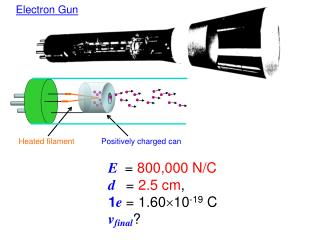DownloadDownload PresentationHeated filament

# Heated filament

Télécharger la présentation## Heated filament

- - - - - - - - - - - - - - - - - - - - - - - - - - - E N D - - - - - - - - - - - - - - - - - - - - - - - - - - -
##### Presentation Transcript

1. Electron Gun Heated filament Positively charged can E = 800,000 N/C d = 2.5 cm, 1e = 1.6010-19 C vfinal?

2. E = 800,000 N/Cd = 2.5 cm, 1e = 1.6010-19 C vfinal? What voltage difference do the electrons experience jumping the gap? How much energy is given to each electron? An electron with this much energy, must be moving how fast?

3. When the electric field is uniform,V = Ed + + + + + + + + + + + + + + + + + + + + + + + + + + + + + + + + + + + + + + - - - - - - - - - - - - - - - - - - - - - - - - - - - - - - - - - - - - - - When the electric field is NOT uniform V  Ed But for an isolated concentration of charge we have

4. Parallel Plate Capacitor • device to storecharge • (also stores energy) • connect capacitor to battery (V) • plates become oppositely charged +Q/-Q +Q -Q A chargepotential difference d Q= C V + - • constant of proportionality is called the capacitance C 12 V

5. The 750mF capacitor in a camera flash attachment is charged up by a 6V battery. What Q does it store? Q=CV = (6 volts)(75010-6 coul/volt) = 4.510-3 coul

6. ConcepTest A one millifarad capacitor has is has a voltage of 100 V across it. What is the total charge on the capacitor? 1) Zero 2) 0.1 C 3) 10 C 4) 100,000 C What is the charge on the positive plate of the capacitor? 1) Zero 2) 0.05 C 3) 0.1 C 4) 0.2 C

7. Capacitors Capacitance depends only on geometry Example: parallel-plate capacitor A = area of plate d = separation between plates • e0 = 8.85 x 10-12 C2/N-m2 (permittivity of free space)

8. How big is 1 Farad? • choose d = 1 mm • find the area A for such a capacitor A =C(d/e0) =(1 F) (0.001 m) /(8.85x10-12) =1.1 x 108 m2 =110 km2 This capacitor is as big as a city!!!

9. Capacitor C1 is connected across a battery of 5 V. An identical capacitor C2 is connected across a battery of 10 V. Which one has the most charge? 1) C1 2) C2 3) both have the same charge 4) it depends on other factors

10. Q= C V What must be done to a capacitor in order to increase the amount of charge it can hold (for a constant voltage)? • 1) increase the area of the plates • 2) increase the separation between the plates • 3) decrease the separation between the plates • 4) decrease the area of the plates • 5) either (1) or (2) • either (1) or (3) • either (2) or (4) • either (3) or (4)

11. Increasing the separation, d, of the two charged plates of a capacitor, after it has been disconnected from its battery, will (1) Increase the charge on the plates. (2) Increase the capacitance of the plates. (3) Increase the voltage across the plates. (4) None of the above.

12. A parallel-plate capacitor initially has a potential difference of 400 V and is then disconnected from the charging battery. If the plate spacing is now doubled (without changing Q), what is the new value of the voltage? 1) 400 V2) 200 V 3) 100 V 4) 800 V 5) 1600 V

13. Inserting a dielectric material between two charged parallel conducting plates, originally separated by air and now disconnected from the battery, will (1) Increase the charge on the plates. (2) Increase the capacitance of the plates. (3) Increase the voltage across the plates. (4) None of the above.

14. Answers to Multiple Choice Questions 2) 0.1 C 3) 0.1 C 2) C2 6) either (1) or (3) (3) Increase the voltage across the plates. 4) 800 V (2) Increase the capacitance of the plates.